# Complex Numbers

A complex number is a number with a real and an imaginary part, usually expressed in cartesian form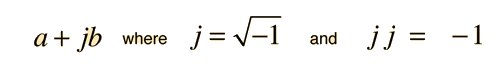a + jb = + j

Complex numbers can also be expressed in polar form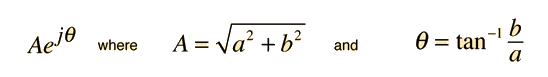A = , θ = radians =°

The polar form can also be expressed in terms of trigonometric functions using the Euler relationship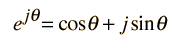Index

Complex numbers

 HyperPhysics****HyperMath*****Algebra R Nave
Go Back

# Euler Relationship

The trigonometric functions are related to a complex exponential by the Euler relationship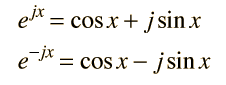From these relationships the trig functions can be expressed in terms of the complex exponential: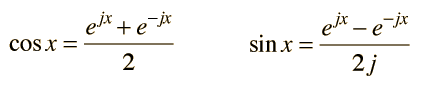This relationship is useful for expressing complex numbers in polar form, as well as many other applications.

Applications:
 Traveling waves
Index

Complex numbers

 HyperPhysics****HyperMath*****Algebra R Nave
Go Back

# Polar Form, Complex Numbers

The standard form of a complex number isbut this can be shown to be equivalent to the formwhich is called the polar form of a complex number. The equivalence can be shown by using the Euler relationship for complex exponentials.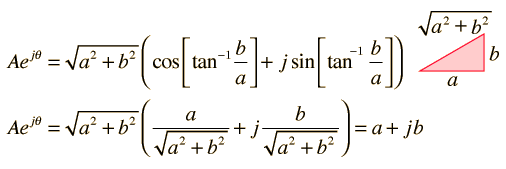Index

Complex numbers

 HyperPhysics****HyperMath*****Algebra R Nave
Go Back